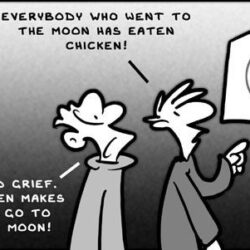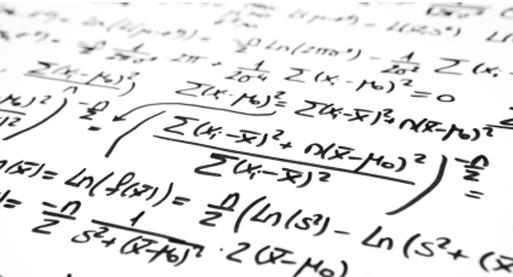# ROC curve-what you always wanted to know but were afraid to ask…

This post is inspired, as many of them, by the student’s question. It appears that many of us use ROC curves in the analysis not really knowing what these curves are for and how they are created. The classification models have become a very popular tool and are part of many implemented widely available black-box Read more about ROC curve-what you always wanted to know but were afraid to ask…[…]# Pairwise comparisons

Post-hoc pairwise comparisons are very common part of the data scientist daily routine. Whenever we do ANOVA or non-parametric testing with more than two groups that is inevitable. At some point you might want to have a nice visualizations of what is significant and what is not. Possibly with p-values written on the plot. Then Read more about Pairwise comparisons[…]# Sankey plots

Sankey plots are often used to illustrate flows, for example treatment changes in medicine. The plot above illustrates the treatment changes in asthma patients. Some small flows are omitted. Thanks to network3D package doing sankey plots has become fun! By implementing java scripts they can be interactive. There are however some traps when using this Read more about Sankey plots[…]# Forestplot R package – what you CAN’T do with it.

When you do meta-analysis you usually visualize your results using a so-called forest plot. The plot is automatically available in the packages denoted for meta-analysis (such as meta), but there are times you would like to plot it “from the scratch” and completely customize it. After struggling a bit with ggplot2 you discover forestplot package Read more about Forestplot R package – what you CAN’T do with it.[…]# Interactions and why we all get them wrong?

Where the interpretation of the interaction comes from? Let us assume that we have patients in out sample and a linear model (simple or mixed). For the linear model we have an equation of the form: Y=A0+A1*X1+A2*X2+A3*X1*X2, where A0 is the intercept and A1 and A2 are the main effects of X1 and X2 respectively. Read more about Interactions and why we all get them wrong?[…]# Few thoughts about sample size calculation for a single proportion

Let’s say we need to calculate a sample size for a single proportion estimation (testing). Omitting now maths and some variability in the used formulas we can do it two in two ways: First: using power of a single test and then we just require the test is rejecting or not the hypothesis of a Read more about Few thoughts about sample size calculation for a single proportion[…]